# What is a Pip in Forex

Maybe you've been in the middle of watching a movie trailer on YouTube, and out of nowhere this ad appears with a guy who tells you how to make money in Forex. The ad gets your attention and you decide to hear this guy out. Then, just as it is getting more and more interesting, the guy starts talking about 100 pips a day. The ad, which at first seemed to be interesting, suddenly confuses you.

You were wondering: what exactly is a pip? And why did he quantify his profits in pips? Don't worry: this article will expand your knowledge about pips!

## So what is a pip in Forex?

A pip is an abbreviation for “point in percentage” and represents the smallest unit of change in the value of a currency pair. For most currencies, especially the majors, a pip represents the fourth decimal place in the exchange rate for the two currencies. However, this decimal place can vary for some currency pairs. For currency pairs that involve JPY, a pip is represented by the second decimal place.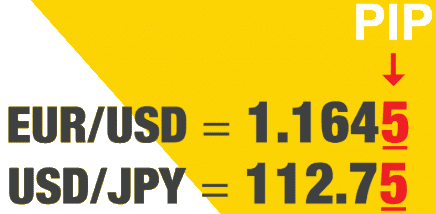Let's take an example. Let's suppose you are a trader who is trading EUR/USD. You opened a long position when the exchange rate was 1.2712. You predicted that the price would go up, and after a few minutes the price moved to 1.2713 and you decided to close your trade. The price change here is 0.0001, which equals 1 pip.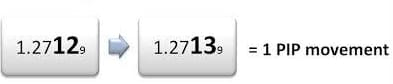Let's take a look at a real market situation. Let's assume that you opened a long position when the price was 1.1438, as shown in the table below. You predicted that the price would go up, but the price is in fact going in the opposite direction. Now you decide to close the position when the exchange rate is 1.1431. So how much did you lose? You have lost the entire change in the value of the currency pair – 0.0007 – which equals 7 pips.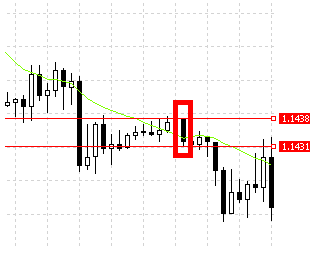## What is a pipette?

The majority of trading platforms use pips as their smallest units of measurement for the change in value of a currency pair. However, the need for more accuracy has led to the introduction of a pipette, which is 1/10 of a pip. In this case, a pipette is represented by the fifth decimal place on your trading platform. When JPY is involved in the currency pair, a pipette is represented by the third decimal place.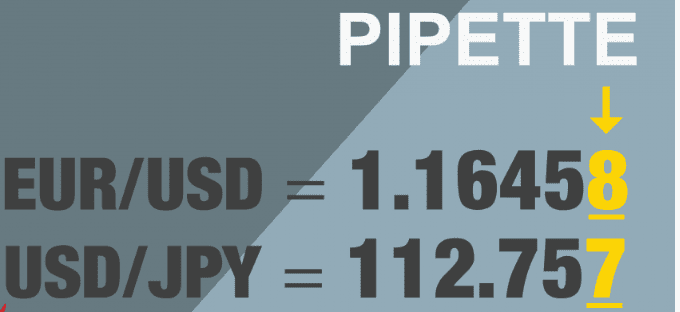Let's use the previous example, but this time with a broker platform that allows the use of pipettes.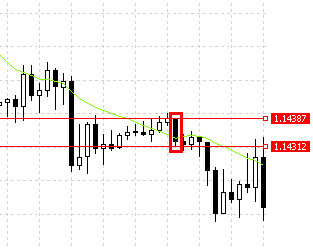In this example, you opened a long position when the exchange rate was 1.14387. You expected that the price would rise. Unfortunately, that was not the case. Instead, the price moved against your position. Now you decide to close your trade at 1.14312. You end up losing 0.00075, which equals 75 pipettes. I know that after looking at this example, you will appreciate the accuracy that pipettes provide. Pipettes provide the trader with a higher degree of accuracy than pips. In the previous example, the loss was 7 pips. But now we get a clearer picture with the more granular unit of measurement: 75 pipettes (7.5 pips).

## The importance of pips in Forex Trading

You use pips to quantify how much you have won or lost on a particular trade.

Expressing your profits or losses in currency amounts can be confusing and difficult to compare. That's because the amount of dollars gained or lost depends on many factors.

A small shift on the market could lead to huge profits, while on the other hand a big market move could result in just a small profit where both are measured in dollars. Thus, pips remain the only reliable way to quantify fluctuations on the market.

## Value of pips

A pip value can be defined as the price attributed to a move by one pip on the foreign exchange market. When you have a long position and the price is moving in your favor, your open trade will increase in value. The open position behaves in a similar way when the price moves against you. The pip value will tell you how much the incremental profit is worth. To get this value, we need to calculate the pip value.

Since the value of a pip is very tiny, Forex is always traded in standard lots, mini lots and micro lots. A standard lot is 100,000 units of the base currency; a mini lot is 10,000 units, while a micro lot is 1,000 units of the base currency. We also have a nano lot, which is 100 units of the base currency. Below you can find a list of how the different lot sizes affect the value of a pip.

Lot size Units of base currency Volume Pip value in USD 1 standard lot 100,000 1.0 1 pip=\$10 1 mini lot 10,000 0.1 1 pip=\$1 1 micro lot 1,000 0.01 1 pip=\$0.1 1 nano lot 100 0.001 1 pip=\$0.01

 Lot size Units of base currency Volume Pip Value in USD 1 standard lot 100,000 1.0 1 pip=\$10 1 mini lot 10,000 0.1 1 pip=\$1 1 micro lot 1,000 0.01 1 pip=\$0.1 1 nano lot 100 0.001 1 pip=\$0.01

## Calculation of the pip value and position size - with examples

As we have already described, the pip value shows how much a pip movement contributes to your profit or loss. The pip value is important, because it helps you to manage risk. For example, if you don't understand the pip value, how can you calculate the ideal position size? So, if you don't understand the concept of the pip value, it will be difficult for you as a trader to measure and manage your risk.

Let's assume that you have a trading account denominated in euros, and you would like to trade 1 standard lot of EUR/USD at the exchange rate of 1.20. In the case of EUR/USD, 1 pip is the same as 0.0001.

Pip value = 0.0001/1.20*100,000 = 8.333 Euro

### Pip value for accounts denominated in USD

Many trading accounts are denominated in US dollars. Whenever the USD is listed second in a currency pair and the account is denominated in US dollars, the pip value does not change.

In such a case, a standard lot has a pip value of \$10; a mini lot has a pip value of \$1; and a micro lot has a pip value of \$0.1. This applies to each currency pair as long as the USD is listed second. Here are some examples: EUR/USD, AUD/USD, GBP/USD, NZD/USD.

If the USD is the base currency (listed first in the currency pair), simply use the formula that was mentioned above. Let's say that you are trading a standard lot of the currency pair USD/CAD. As you can see, the USD is listed first in this case. Assuming that the exchange rate of USD/CAD is 1.25, the pip value in US dollars would be 10/1.25 = \$8. Below you can see how to calculate the pip value for mini lots and micro lots.

Pip value for standard lots = 10/ (USD/XXX)

Pip value for mini lots = 1/ (USD/XXX)

Pip value for micro lots = 0.1/ (USD/XXX)

### Pip value for accounts not denominated in USD

Let's assume you have an account denominated in Canadian dollars. Each time you trade a currency pair with the Canadian dollar listed second, the pip value remains fixed. In such a case, a standard lot has a pip value of CAD\$10; A mini lot has a pip value of CAD\$1; and a micro lot has a pip value of CAD\$0.1.

What happens if the Canadian dollar is listed first, like in the case of CAD/CHF? You get the pip value by dividing the fixed rates from above by the exchange rate. Let's assume the exchange rate of CAD/CHF is 0.8. So what is the pip value for a micro lot? It will be CAD\$0.1/0.8 = CAD\$0.125. You can do the same for standard lots and mini lots.

Pip value for standard lots = 10/ (CAD/XXX)

Pip value for mini lots = 1/ (CAD/XXX)

Pip value for micro lots = 0.1/ (CAD/XXX)

What if the currency pair now has CAD as the base currency and JPY as the quoted currency (CAD/JPY)? Let's show an example: Let's say the exchange rate for CAD/JPY is 90.00. What would be the pip value for a standard lot in this case?

We will use the formula discussed above, but will then multiply the result by 100.

You can use this process for other currencies like EUR or even the Australian dollar.

### The pip value for other currency pairs

Maybe you have an account denominated in USD, but you are trading a currency pair that does not include the US dollar. Maybe you have an account denominated in USD, but you have chosen to trade a currency pair like EUR/CHF or EUR/GBP.

Let's take the example EUR/CHF. The established rule is that if you have an account denominated in CHF and you are trading EUR/CHF, then the pip value is fixed (CHF 10 for standard lots, CHF 1 for mini lots and CHF 0.1 for micro lots)

In this case, let's assume that we calculate the pip value for a standard lot, which is fixed at CHF 10. So if my account were denominated in USD, I would get my pip value by dividing CHF10/(USD/CHF). This is the fixed value divided by the USD/CHF exchange rate. If the exchange rate of USD/CHF is, for example, 0.80, the pip value would be 10/0.80 = USD 12.50.

What would happen if you couldn’t find the rate for USD/CHF and instead found the rate for CHF/USD? What would you do in that situation?

You must take the inverse rate of CHF/USD to get the rate for USD/CHF. Let's say that you found that the rate for CHF/USD is 1.25. In that case, the inverse rate would be 1/1.25 = 0.80.

## Changes in the pip value

In most cases, the base currency of your account will determine the pip value of the various currency pairs. If your account is denominated in USD and the currency has USD as the quoted currency (the one that is listed second in the currency pair), for example  EUR/USD, then the pip value will be fixed as we discussed earlier. In such a case, a standard lot has a pip value of \$10; a mini lot has a pip value of \$1; and a micro lot has a pip value of \$0.1.

A change in the pip value will only occur if the exchange rate of the US dollar were to move by more than 10%, while the USD is the base currency (for example, USD/CAD or USD/JPY) or the USD is not included in the currency pair (for example GBP/JPY). The account is denominated in USD.

A good example is when the exchange rate for USD/JPY fell from about 120 to a low of about 77 between 2008 and 2011. The rapid strengthening of the Yen caused the pip value for the currency pair to change. In this case, the movements on the market had a significantly greater effect on value as the pip value rose.

Based on the knowledge that we gained, let's see now what effect the change had on the pair's pip value. The exchange rate moved in this case from 120 to 77. Prior to 2008, the pip value for standard lots of USD/JPY on an account denominated in USD was \$10/120 * 100 = 8,333. By 2011 the exchange rate moved to 77 and the pip value rose during the period to \$10/77 * 100 = 12.98. Therefore, the market movements had a greater effect on value.

## The relevance of pip values while hedging

Hedging involves the simultaneous purchase and sale of securities to reduce risk. Many traders see this as a risk-free position, as losses on the one hand are offset by profits on the other hand. However, this is not always the case. Hedging does entail a certain amount of risk, as wide spreads can eat into both positions, which can result in losses.

The widening of spreads mainly occurs in times of important global events, such as the moment when the Swiss National Bank scrapped the 1.20 francs per euro cap back in 2015. Brexit is another major global event, which may hurt your hedged trades.

During such times the spread fully depends on offers and demands. The spread can even be 100 pips wide. If that happens to both of your positions, the results may be devastating. If the currency pairs involved are illiquid, the spreads are likely to be even wider, which would lead to more losses for the hedged position.

## What is a pip for CFDs?

Before we get to the point of discussing pips in CFDs, let's talk first about some important things. What is a CFD? A CFD is a contract that allows a trader to trade and to take advantage of the price movements of the underlying assets without actually owning them.

So are there pips in CFD trading? The term is not often used in CFD trading. Instead, there are terms like cents and pence.

Let's say the price of a CFD is, for example, \$1.00. If the price moves to \$1.01, we can say that it rose by 1 cent. The price in cents is always to the right of the decimal point, while on the left you can see the price in USD.

## Summary

As a result of reading this article, you should now understand that a pip is the smallest unit of price change that is measurable for a currency pair. You also now know about the crucial role that the pip value plays in trading. In the course of your actual trading, you don't need to calculate the pip value by yourself, as there are some calculators to do this job for you. To learn more, you should register a demo account so that you can experience how the pip value may affect your profits.

## Why should you trade Forex with Libertex?

Founded in 1997, Libertex is an international, trusted brand with more than 20 years of experience in financial markets and online trading, serving clients in more than 110 countries. Every year, the company consolidates its leading position both in the markets where it is already present and in new markets that it enters with its innovative products and services.

• Licensed and regulated CFD broker
• 2.2 million clients worldwide
• 27 countries covered
• 30 international awards